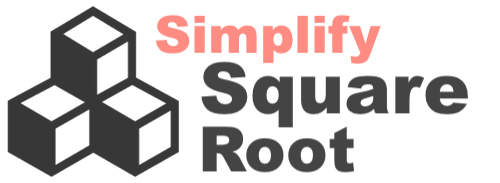Simplify Square Root of 12346What is the square root of 12346 in its simplest radical form? To be more specific, we have created an illustration below showing what we want to calculate. Our goal is to make "A" outside the radical (√) as large as possible, and "B" inside the radical (√) as small as possible.

12346 = A√B

Unfortunately, the square root of 12346 cannot be simplified. Thus, here is the answer to the square root of 12346 in its simplest form:

12346 = √ 12346

Here are two different methods we used to determine why the square root of 12346 cannot be simplified.

1) To be able to simplify the square root of 12346, one of the factors of 12346 other than 1 must be a perfect square. The factors of 12346 are 1, 2, 6173, and 12346. Since none of these factors are perfect squares, the square root of 12346 cannot be simplified.

2) To be able to simplify the square root of 12346, all the prime factors of 12346 cannot be unique. When we did prime factorization of 12346, we found that 2 x 6173 equals 12346. Since all the prime factors are unique, the square root of 12346 cannot be simplified.

Simplify Square Root
Please enter another square root in the box below for us to simplify for you.

Simplify Square Root of 12347
Here is the next square root on our list that we have simplifed.